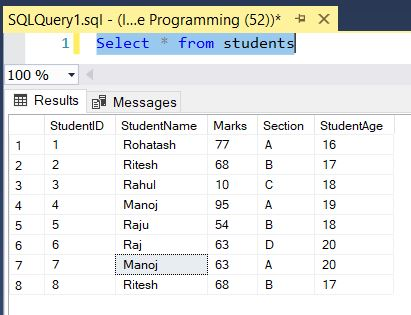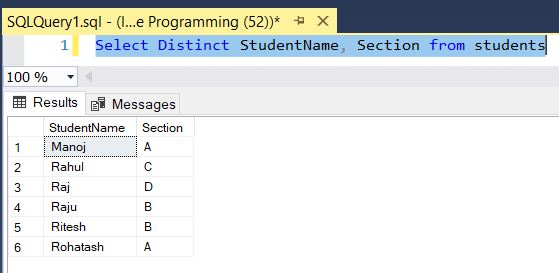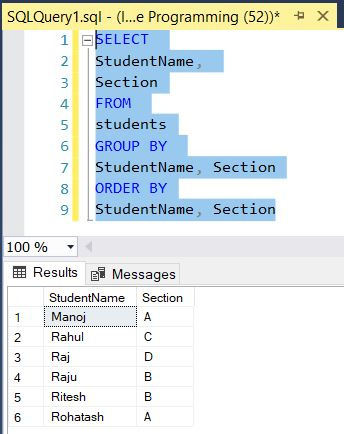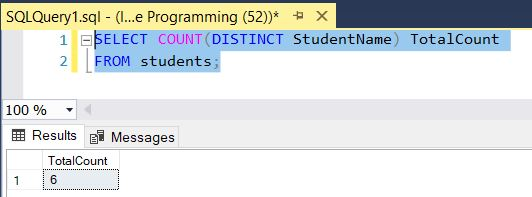﻿ SQL Distinct - TutorialsTrend
This Angular tutorial helps you get started with Angular quickly and effectively through many practical examples.
`

# SQL-Distinct Keyword

#### SQL SELECT DISTINCT

The SQL DISTINCT keyword is used to retrieve only distinct or unique data. In a table, If a table have some duplicate value and sometimes we want to retrieve only unique values. In such case, SQL SELECT DISTINCT statement is used.

To do this, you use the SELECT DISTINCT clause as below:

``````SELECT DISTINCT
column_name1,
column_name2 ,
...
FROM
table_name;``````

Now create a table named students and insert some record with unique and duplicate. To find list of all table records:

``Select * from students``

Query output looks like that:#### Select DISTINCT example

This statement returns all Student name and section from all students:

``Select Distinct StudentName, Section from students``

Query output looks like that:#### DISTINCT vs GROUP BY Clause

Both DISTINCT and GROUP BY clause reduces the number of returned rows in the result set by removing the duplicates. The following statement uses the GROUP BY clause to return distinct student name together with section from the students table:

``````SELECT
StudentName,
Section
FROM
students
GROUP BY
StudentName, Section
ORDER BY
StudentName, Section``````

The following figure shows the output:#### DISTINCT with COUNT

If we need to count the number of unique rows, we can use the SQL COUNT() function with the SELECT DISTINCT statement.

``````SELECT COUNT(DISTINCT StudentName) TotalCount
FROM students;``````

The following figure shows the output:Next